The GENMOD Procedure

Type 3 Analysis

A Type 3 analysis is similar to the Type III sums of squares used in PROC GLM, except that likelihood ratios are used instead of sums of squares. First, a Type III estimable function is defined for an effect of interest in exactly the same way as in PROC GLM. Then maximum likelihood estimates are computed under the constraint that the Type III function of the parameters is equal to 0, by using constrained optimization. Let the resulting constrained parameter estimates be
and the log likelihood be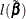. Then the likelihood ratio statistic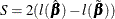where
is the unconstrained estimate, has an asymptotic chi-square distribution under the hypothesis that the Type III contrast is equal to 0, with degrees of freedom equal to the number of parameters associated with the effect.

When a Type 3 analysis is requested, PROC GENMOD produces a table that contains the likelihood ratio statistics, degrees of freedom, and p-values based on the limiting chi-square distributions for each effect in the model. If you specify either the DSCALE or PSCALE option in the MODEL statement, F statistics are also computed for each effect.

Options for handling the dispersion parameter are the same as for a Type 1 analysis. The dispersion parameter can be specified to be a known value, estimated from the deviance or Pearson’s chi-square divided by degrees of freedom, or estimated by maximum likelihood individually for the unconstrained and constrained models. By default, PROC GENMOD estimates scale by maximum likelihood for each model fit.

The results of this type of analysis do not depend on the order in which the terms are specified in the MODEL statement.

A Type 3 analysis can consume considerable computation time since a constrained model is fitted for each effect. Wald statistics for Type 3 contrasts are computed if you specify the WALD option. Wald statistics for contrasts use less computation time than likelihood ratio statistics but might be less accurate indicators of the significance of the effect of interest. The Wald statistic for testing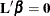, where
is the contrast matrix, is defined by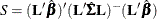where
is the maximum likelihood estimate and
is its estimated covariance matrix. The asymptotic distribution of S is chi-square with r degrees of freedom, where r is the rank of.

For models that use less-than-full-rank parameterization (as specified by the PARAM=GLM option in the CLASS statement), a Type 3 test of an effect of interest (main effect or interaction) is a test of the Type III estimable functions that are defined for that effect. When the model contains no missing cells, the Type 3 test of a main effect corresponds to testing the hypothesis of equal marginal means. For more information about Type III estimable functions, see Chapter 46: The GLM Procedure, and Chapter 15: The Four Types of Estimable Functions. Also see Littell, Freund, and Spector (1991).

For models that use full-rank parameterization, all parameters are estimable when there are no missing cells, so it is unnecessary to define estimable functions. The standard test of an effect of interest in this case is the joint test that the values of the parameters associated with that effect are zero. For a model that uses effects parameterization (as specified by the PARAM=EFFECT option in the CLASS statement), the joint test for a main effect is equivalent to testing the equality of marginal means. For a model that uses reference parameterization (as specified by the PARAM=REF option in the CLASS statement), the joint test is equivalent to testing the equality of cell means at the reference level of the other model effects. For more information about the coding scheme and the associated interpretation of results, see Muller and Fetterman (2002, Chapter 14).

If there is no interaction term, the Type 3 test of an effect for a model that uses GLM parameterization is the same as the joint test of the effect for the model that uses full-rank parameterization. In this situation, the joint test is also called the Type 3 test. For a model that contains an interaction term and no missing cells, the Type 3 test of a component main effect under GLM parameterization is the same as the joint test of the component main effect under effect parameterization. Both test the equality of cell means. But this Type 3 test differs from the joint test under reference parameterization, which tests the equality of cell means at the reference level of the other component main effect. If some cells are missing, you can obtain meaningful tests only by testing a Type III estimation function, so in this case you should use GLM parameterization.

The results of a Type 3 test or a joint test do not depend on the order in which you specify the terms in the MODEL statement.

Generalized score tests for Type III contrasts are computed for GEE models if you specify the TYPE3 option in the MODEL statement when a REPEATED statement is also used. See the section Generalized Score Statistics for more information about generalized score statistics. Wald tests are also available with the Wald option in the CONTRAST statement. In this case, the robust covariance matrix estimate is used forin the Wald statistic.